# Advantages of Computer Programs Over Power Charts for the Estimation of Power

Guido G. Gatti and Michael Harwell
University of Pittsburgh

Journal of Statistics Education v.6, n.3 (1998)

Copyright (c) 1998 by Guido G. Gatti and Michael Harwell, all rights reserved. This text may be freely shared among individuals, but it may not be republished in any medium without express written consent from the authors and advance notification of the editor.

Key Words: Noncentrality; Software; Statistics textbooks; Student learning.

## Abstract

Statistics and research design textbooks routinely highlight the importance of a priori estimation of power in empirical studies. Unfortunately, many of these textbooks continue to rely on difficult-to-read charts to estimate power. That these charts can lead students to estimate power incorrectly will not surprise those who have used them, but what is surprising is that textbooks continue to employ these charts when computer software for this purpose is widely available and relatively easy to use. The use of power charts is explored, and computer software that can be used to teach students to estimate power is illustrated using the SPSS and SAS data analysis programs.

# 1. Introduction

1 The importance of estimating the power of a statistical test to reject a null hypothesis has received extensive attention in several substantive research literatures (e.g., applied statistics, education, psychology, and nursing). One of the earliest articles on this topic was by Cohen (1962), who documented a lack of concern toward power among researchers. Despite attention to this topic by methodologists in the quantitative research literature (e.g., Brewer 1972; Dayton, Schafer, and Rogers 1973), concern over power has not abated (Thomas and Krebs 1997, pp. 128-139).

2 Statistics and research design textbooks reflect the attention given to this topic through their emphasis on a priori estimation of power (e.g., Glass and Hopkins 1984; Hays 1994; Keppel 1991; Kirk 1995; Maxwell and Delaney 1990). One thing these and other textbooks share is that each presents techniques for estimating power using the charts given in Pearson and Hartley (1951). Pearson and Hartley expanded Tang's (1938) tables for estimating power of the analysis of variance (ANOVA) F test but retained Tang's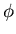parameter (defined below) and nominal Type I error rates of .05 and .01 in their charts. That the use of power charts can lead to estimating power incorrectly will not surprise those who have used them. The problem is exacerbated by reprinting the charts in textbooks in even smaller print than that used in the original publication. We argue that teaching students to estimate power using these charts is undesirable because they are difficult to use and unnecessary because of the availability of relatively easy-to-use computer software designed for this task.

3 Teaching students to estimate power using the Pearson and Hartley charts sets the stage for several difficulties. The likelihood that students will estimate power incorrectly because of the difficulty of separating one curve from another in these charts or because of the interpolation that is often necessary seems quite high. This perception has been reinforced by our observation that even students who have grasped the ideas underlying statistical power frequently estimate power incorrectly using these charts. (The results of a small empirical study reported later supports this perception.) Students are also likely to be confused if, after entering the charts with the correct parameters, they obtain answers that differ from those of their peers or those given in the textbook. This unhealthy state of affairs motivated us to look for alternative approaches to estimating power, which led us to the introductory statistics textbook by Moore and McCabe (1993). These authors recommended the use of the SAS (SAS Institute Inc. 1990) data analysis program to estimate power for the simple reason that the estimates given by SAS are not subject to the difficulties associated with the power charts. We concur with this recommendation, although we recognize that others may prefer different software.

4 We begin by defining power and the associated concepts of a noncentrality parameter and a noncentral distribution. We focus on the single factor, fixed effects, completely randomized between-subjects ANOVA model and assume that the design is balanced and that the statistical assumptions underlying the F test are satisfied. However, power can be estimated for other ANOVA models and for other statistical tests (see, e.g., Odeh and Fox 1991). Our presentation of power and related examples focuses on the textbook by Kirk (1995), which we selected because its coverage of power is one of the most comprehensive we know of among statistics and research design textbooks. Still, we emphasize that our comments and criticisms apply to many textbooks and that we are using Kirk's (1995) textbook as an example. We remind readers that estimating power and sample size are intertwined, and that estimating power for a given sample size and treatment effect, and estimating sample size for a specified power and treatment effect, are two sides of the same statistical coin. Our focus is on estimating power for specified sample sizes and treatment effects.

5 If the null hypothesis of equal population means in an ANOVA model is true, then the associated F statistic has a central F distribution with two parameters, the numerator () and denominator (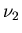) degrees of freedom. But if the null hypothesis is false, the F statistic has a noncentral F distribution that depends onandand a noncentrality parameter, defined as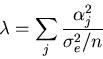,

where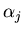=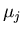-and represents a treatment effect,is the mean of the jth population,is the grand mean,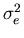is the population error variance common to the j = 1, ..., p populations, and n is the common size of the samples from the p populations. Since= 0 in the null case and does not equal 0 in the non-null case,reflects the magnitude of the treatment effect. Under the assumption that the observations are independent, normally distributed, and homoscedastic, power (the probability of rejecting a false null hypothesis) is computed by referring to a noncentral F distribution with values for the above parameters and the nominal Type I error rate ().

# 2. Difficulties With the Pearson and Hartley Power Charts

6 Once students have mastered the theory behind statistical power, the next step is to have them estimate power using real or contrived data. Most statistics and research design textbooks with which we are familiar teach students to estimate power using the Pearson and Hartley charts. Students using the Kirk (1995) text learn to estimate power by computing the parameter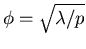, and entering the Pearson and Hartley charts in the back of the text with specified values for,,, and. Using this information, students select the appropriate curve based onand the denominator degrees of freedom. As students quickly learn, interpolation is typically necessary since the exact denominator degrees of freedom will often not be represented by a specific curve.

7 For example, for p = 4 groups and a sample size of n = 5 from each population, the denominator degrees of freedom are 16. However, there is no curve corresponding to 16 degrees of freedom on p. 818 in Kirk (1995) (15 is the nearest value). Another shortcoming of the power charts is that they are only provided for nominal Type I error rates of .01 and .05. Although these are standard values, there are settings in which estimating power for othervalues may be important. For example, suppose that three (orthogonal) a priori comparisons are specified and that= .05 is divided equally among the comparisons to be tested. Estimating power for the comparisons is difficult because a curve corresponding to theper comparison value of 1 - (1 - .05)1/3 = .017 does not appear in the charts.

8 Nor is it possible to estimate power forvalues less than one. For example, if= 2,= 60, and<1 it is only possible to conclude that power < .31 since the smallestvalue in the charts is one.

9 Before continuing, we note that estimating power incorrectly using the Pearson and Hartley charts produces either over- or underestimates that can affect the statistical analysis and subsequent conclusions. Overestimates lead to statistical tests possessing less than the desired power, since researchers would be using a smaller sample size than would be necessary to ensure the desired power. For example, suppose a researcher wanted to ensure that a statistical test had a minimum power of .80 to detect a specified treatment effect for a givenand sample size. Suppose also that the use of the power charts yielded an estimated power of .80 for a sample size of n, but that the true power was .76. The result is that the statistical test would have less than the desired power. Underestimates, on the other hand, may lead to the use of larger samples than is necessary, which may be costly and inefficient.

10 Some readers may feel that only moderate or large instances of estimating power incorrectly are serious, and that small errors of estimation using the power charts can safely be ignored or dealt with by simply increasing the sample size per group by one or two beyond the n indicated by the sample size and power calculations. Our view is that even small estimation errors (e.g., 2-3%) may be important. One reason is that even small estimation errors may confuse students trying to master this concept. Another is that the magnitude of treatment effects is often quite modest, making it imperative that statistical tests possess the desired power to detect such effects. Under these conditions, even small estimation errors can lead to lower than desired power and an unacceptably high probability that effects of interest will not be detected. Nor can it be assumed that increasing each sample by one or two is always feasible, because of, for example, resource constraints.

# 3. Estimating Power

11 Kirk (1995) describes three methods of estimating power that are distinguished by the amount of information users must specify; however, all methods require that,,, andbe specified. As in many statistics and research design textbooks, Kirk (1995) uses data to illustrate the estimation of power. It is useful at this point to distinguish between prospective power, which represents the probability of rejecting a false null hypothesis before data are collected, and retrospective power, which is the probability of rejecting a false null hypothesis after data have been collected and the associated null hypothesis has been rejected. (Estimating power for already-collected data for which the null hypothesis was retained has no meaning). Zumbo and Hubley (1998) point out that the probabilities representing prospective and retrospective power for a given problem are not necessarily the same, and that estimation of prospective power is preferred. Unfortunately, Kirk's (1995, p. 183) example and those in many other textbooks describe the data as coming from a pilot study or an actual study, meaning that retrospective power is being estimated. It is important to emphasize to students that power should be estimated prospectively.

12 For the example presented in Kirk (1995, p. 183) with p = 4 and= .05, .80 is given as the minimally acceptable power. For n = 8, Kirk (1995) estimatesandusing the pilot data as:= 5.308/(2.167/8) = 19.6,= (19.6/4).5 = 2.21. The question being asked is, for these values, what is the power to reject the null hypothesis under consideration? Entering the Pearson and Hartley power charts with= 2.2,= p - 1 = 3 and= p(n - 1) = 28, the estimated power is given as .95. Kirk (1995) also shows how to estimate power using Cohen's f and the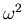(omega squared) measure of explained variation (Cohen 1988).

13 Estimating power using the Pearson and Hartley charts as described in Kirk (1995) is one way for students to learn to estimate power; another is for students to use computer software to estimate power. These programs fall into one of three categories: programs devoted to power and sample size estimation, internet web sites that can be used to estimate power, and general purpose data analysis programs that can be used to estimate power as well as perform various statistical analyses. Thomas and Krebs (1997) provide a review of 29 programs that can be used to calculate power or sample size; 13 of these are stand-alone power and sample size programs. The second category is populated by internet sites. Thomas and Krebs (1997) also provide addresses of internet sites that are dedicated to estimating power. These sites function much like the software dedicated to estimating power in that users need only submit a few pieces of information to have a power value returned. There are also a number of sites in which probability calculators can be used to estimate power if the necessary parameter values are submitted (e.g.,). An example of this kind of site is the interactive probability calculator hosted by UCLA's Department of Statistics (http://www.stat.ucla.edu/calculators/cdf/). Popular statistical packages that fall into the third category are SPSS's Sample Power and SAS. Sample Power is currently a separate module that may appear in a future version of SPSS for Windows (personal communication, SPSS Inc.). A 30-day evaluation copy of Sample Power can be obtained from http://www.spss.com/software/spower/. Users can also use the General Linear Model dialog box in the main SPSS program to estimate power; however, these calculations can only be done with data, implying that the SPSS General Linear Model program is generally estimating retrospective power. While these programs have much to recommend them, we use SAS to estimate power as recommended by Moore and McCabe (1993) because of its versatility, familiarity, and availability to many students. However, we illustrate the use of both SAS and the SPSS program Sample Power to estimate power.

14 SAS can be used to estimate power through the probability functions in its IML procedure (SAS Institute Inc. 1990), a module integrated into the SAS program. In general, two functions are called, one (FINV) that provides the corresponding F value for specified,, andvalues (although users could look these up), and another (PROBF) that is used to compute power. SAS/IML usesas the noncentrality parameter, which is easily obtained as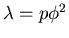. The SAS commands to compute power and to print this value are

     PROC IML;
F = FINV(PR, DF1, DF2, 0);
POWER = 1 - PROBF(F, DF1, DF2, NCP);
PRINT 'F VALUE = ' F;
PRINT 'POWER = ' POWER;


where DF1 =, DF2 =, PR = 1 -, and NCP =represents the noncentrality parameter. The function FINV returns the F value for the specified parameters (0 returns the central F distribution). The PROBF function returns the probability of retaining a false null hypothesis. Subtracting this value from one gives the power. The FINV and PROBF are internal SAS functions, whereas F and POWER are user-defined variables. This code is the same for both mainframe and PC versions of SAS.

15 These two functions are used to compute the power for Kirk's (1995) example on p. 183 in which= 3,= 28,= .05, and= 19.6. Inserting the parameters from Kirk's (1995) example,

     F = FINV(.95, 3, 28, 0)
POWER = 1 - PROBF(F, 3, 28, 19.6)


SAS returns a power value of .9479608. Rounded off to two decimal places, this is the same as that reported in Kirk (1995) using the power charts. The fact that any parameters may be inserted into the program and an exact value returned eliminates the problems associated with the power charts. Prospective or a priori estimation of power will often require that the above process be repeated for different sample sizes, different numbers of groups, and even different nominal Type I error rates.

16 It is also possible to calculate the power of other statistical tests using the IML procedure. The following pairs of functions can be used to calculate power for tests that require the normal, chi-square, or t sampling distributions:

     PROBIT(PR, 0) PROBNORM(PROBIT, NCP),
CINV(PR, DF, 0) PROBCHI(CINV, DF, NCP),
TINV(PR, DF, 0) PROBT(TINV, DF, NCP),


For a normal distribution (e.g., z test), use PROBIT(PR, 0) and PROBNORM(PROBIT, NCP); for tests with a chi-square distribution, use CINV(PR, DF, 0) and PROBCHI(CINV, DF, NCP); for tests with a t distribution, use TINV(PR, DF, 0) and PROBT(TINV, DF, NCP).

17 SPSS provides a menu-driven alternative to SAS in its Sample Power program, which is closely tied to Cohen's (1988) book. Users may estimate power for various tests (e.g., single- and multi-factor ANOVA and ANCOVA, t-test for a correlation coefficient), effect sizes,values, and sample sizes. Users can also estimate power for tests and designs not included in its menus through the use of non-central t, F, and chi-square probability calculators. These calculators can be accessed under

File >> New Analysis >> General.

To estimate power using Sample Power for the Kirk (1995) example, choose the option Non-central F (ANOVA) and enter .05 for, 3 for df1, 28 for df2, and 19.6 for the noncentrality parameter. When the appropriate parameters are entered, click on Compute. For these parameters, Sample Power returns a power of .95, the same as the value reported in Kirk (1995). Sample Power will also generate tables and graphs of power values for a range of sample sizes and effect sizes. Noncentral t, F, and chi-square probability calculators can also be indexed using the standard SPSS for Windows program under the COMPUTE option, although the SPSS Data Editor must contain data for the calculators to be used (i.e., the probability calculators will not work unless a datafile is open). We reiterate that our preference for SAS is a personal one, and that SAS and SPSS both offer easy ways to estimate power.

# 4. Examples of Estimating Power

18 We suggested earlier that discrepancies are likely to exist between power values estimated using the Pearson and Hartley charts and those estimated by SAS. We examined this possibility in two ways. First, we compared power values reported in three statistics textbooks (Glass and Hopkins 1996; Keppel 1991; Kirk 1995) for various experimental designs and values computed by SAS for the same parameters. Discrepancies between corresponding power estimates provides evidence about estimating power incorrectly using the Pearson and Hartley charts. Implicit in this comparison is that the power values reported in the texts are not typographical errors. We also considered cases in which power could not be estimated using the power charts because of smallvalues. Second, we conducted a small empirical study in which the performance of students learning to estimate power via the Pearson and Hartley charts was evaluated.

## 4.1 Comparing Power Values Estimated Using Statistics Texts And SAS

19 Table 1 reports power values estimated in three statistics textbooks and those generated by SAS. For example, the first line in Table 1 shows that the example given in Glass and Hopkins (1996) for an ANOVA reported a value of .65 using the power charts, whereas SAS returned a value of .688, resulting in a discrepancy of .038. In general, the same pattern of results emerged for the three texts. The medians (.012, .004, .004) and means (.016, .016, .01) of the discrepancies were similar for the Glass and Hopkins (1996), Keppel (1991), and Kirk (1995) texts, respectively. Multiplying these statistics by 100 yields the average discrepancy expressed as a percent. For example, for the Glass and Hopkins (1996) text, the average of the discrepancies between the reported and SAS-generated power values equals .016 or, equivalently, 1.6%. The standard deviation for the Keppel (1991) text was noticeably larger (.041) than those for the Glass and Hopkins (1996) (.016) and Kirk (1995) (.012) texts. Although the average estimation error using the power charts was quite small, all three texts produced some surprising estimation errors. (Keppel (1991, pp. 84-86) cited several computer programs available to estimate power but used the Pearson and Hartley charts.) From an instructional standpoint, these estimation errors are troubling because their number and magnitude have probably been minimized by the expertise of whoever generated the values (presumably the authors), an expertise that students are unlikely to possess. We encourage readers to conduct similar analyses (perhaps with their students!) to explore patterns of discrepancies.

Table 1. Comparing Power Estimated in Three Statistics Texts Using the Pearson and Hartley Charts and SAS

Text Page Prob.# df1 df2 DesignText Power SAS Power |diff|
GH 408 - 2 117 CR 1.72 8.88 .65 .688 .038
GH 408 - 1 117 CR 2.11 8.90 .84 .841 .001
GH 408 - 1 118 CR 2.58 13.31 .975 .951 .024
GH 409 - 2 77 CR 1.83 10.05 .80 .801 .001
GH 409 - 1 98 CR 1.77 6.27 .71 .698 .012
GH 409 - 1 198 CR 2.5 12.50 .94 .940 .000
GH 419 12 3 60 CR 1.53 9.36 .60 .633 .033
KEP 79 - 3 60 CR 1.58 9.99 .74 .729 .011
KEP 79 - 3 92 CR 1.94 15.05 .90 .905 .005
KEP 80 - 3 36 CR 1.25 6.25 .33 .489 .159
KEP 80 - 3 56 CR 1.53 9.36 .68 .696 .016
KEP 80 - 3 76 CR 1.77 12.53 .84 .837 .003
KEP 80 - 3 96 CR 1.98 15.68 .92 .918 .002
KEP 80 - 3 116 CR 2.16 18.66 .96 .959 .001
KEP 91 4a 4 30 CR 1.73 14.96 .83 .830 .000
KEP 91 4b 4 35 CR 1.85 17.11 .90 .889 .011
KEP 91 4c 4 45 CR 2.06 21.22 .84 .845 .005
KEP 91 4c 4 50 CR 2.17 23.54 .90 .897 .003
KEP 91 5a 7 64 CR 1 8 .45 .453 .003
KEP 91 5b 7 64 CR 1.31 13.73 .72 .724 .004
KEP 227 - 1 120 CR 1.99 15.84 .80 .797 .003
Kirk 183 - 3 28 CR 2.21 19.54 .95 .947 .003
Kirk 203 2 2 27 CR 1.55 7.21 .60 .617 .017
Kirk 358 7 4 12 LS 2.26 25.54 .72 .723 .003
Kirk 361 19 3 19 GLS 1.32 6.97 .50 .495 .006
Kirk 362 22 1 8 CO 1.99 7.92 .69 .694 .004
Kirk 401 - 4 36 CRF 1.78 15.84 .85 .863 .013
Kirk 432 5 1 16 CRF 1.75 6.13 .64 .643 .003
Kirk 432 5 1 16 CRF 2.13 9.07 .76 .807 .047
Kirk 583 15 2 15 SPF 1.85 10.27 .75 .737 .013
Kirk 583 15 2 30 SPF 2.70 21.87 .98 .984 .004
Kirk 583 19 2 12 SPF 2.32 16.15 .89 .894 .004
Kirk 583 19 1 12 SPF 2.26 13.73 .92 .925 .005
Kirk 583 19 2 12 SPF 1.88 10.60 .73 .728 .002

NOTE: GH = Glass and Hopkins (1996), KEP = Keppel (1991), prob.# = problem number, CR = completely randomized, LS = latin square, GLS = Graeco latin square, CRF = completely randomized factorial, CO = crossover design, SPF = split-plot factorial with between- and within-subjects effects. |diff| = absolute value of the differences.= .05 in all cases.

20 Another source of inaccuracy occurs for smallvalues. For example, Kirk (1995, p. 206) reports the estimated power as <.35 for a completely randomized design with= 4,= 45 and= .84. However, SAS returns a power of .258 for these parameters. Similarly, power is reported as <.30 on p. 336 in Kirk (1995) and <.40 on p. 400, leading to estimation errors of .175 and .271, respectively. Although some readers may characterize this limitation of the power charts as irrelevant because they believe low power values are unimportant, we can think of at least two reasons why it may be useful to estimate small power values. One is the instructional value of encouraging students to construct and evaluate changes in power curves as a function of changes in effect size and sample size. This practice requires that power for smallvalues be estimated. For example, we have found it valuable for students to see the effect on power of a wide range ofvalues, and we ask students to estimate (retrospective) power for effect sizes and sample sizes reported in published studies that rejected the associated null hypothesis. Both of these exercises frequently produce smallvalues that do not appear in the power charts. A second reason is that researchers sometimes wish to retain null hypotheses in cases where treatment effects are small, given that what constitutes a small treatment effect has been specified by the investigator. This idea is mentioned briefly in Keppel (1991, p. 90) and is described in more detail in Greenwald (1975) and Serlin and Lapsley (1985). Specifying small treatment effects that are declared to be consistent with the null hypothesis entails knowing the power to detect such effects, which in turn requires the use of smallvalues. Again, these estimates cannot be generated using the power charts but are easily returned by SAS or SPSS.

## 4.2 Comparing Power Values Estimated by Students Using the Pearson and Hartley Charts and SAS

21 We also conducted a simple study to examine the accuracy shown by students learning to compute power via the power charts. Forty-five graduate students in a second-semester statistics class taught in a School of Education were given a homework exercise in which they were expected to estimate power using the Pearson and Hartley charts. The instructor (neither of the authors) used the Glass and Hopkins (1996) text. In the assignment, students were given(.05), the number of groups (four), and the sample size for each group (nine) in a single-factor, completely randomized design and asked to estimate power for three noncentrality patterns. In Pattern 1, the two smallest and two largest means were clustered at the most extreme points of the range. In Pattern 2, the four means were evenly spaced along the range. In Pattern 3, means one and four were at the endpoints while means two and three were in the middle of the range. Pattern 1 produced= 1.7, Pattern 2 produced= 1.26, and Pattern 3 produced= 1.2. The exact power values calculated using SAS were .763 for Pattern 1, .482 for Pattern 2, and .442 for Pattern 3. The difference between estimated and exact power values was tabulated for each student and noncentrality pattern.

22 Histograms of the estimation errors of the students for the three noncentrality patterns are shown in Figures 1, 2, and 3. The figures are similar and show that the estimation errors for most students are less than .05, although there are some students who show substantially greater errors. The medians (.013, .018, .018), means (.028, .028, .029) and standard deviations (.047, .030, .029) of the estimation errors for each noncentrality pattern are similar. In fact, the estimation errors for the student data are similar to those reported in Table 1. For noncentrality Pattern 1, the percentages of students who showed discrepancies of at least .05, .04, and .03 were 18%, 20%, and 20%, respectively; for noncentrality Pattern 2, these percentages were 16%, 23% and 36%, respectively; for noncentrality Pattern 3, these percentages were 11%, 23%, and 36%, respectively. It is important to emphasize that the misestimation of power was not confined to a handful of students. Overall, approximately two-thirds of the students made an error of at least .03 for at least one noncentrality pattern, and almost half made an error of at least .03 for at least two noncentrality patterns.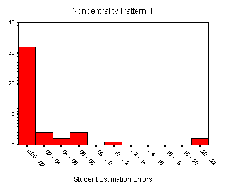Figure 1 (4.0K gif)

Figure 1. Histogram of Student Estimation Errors for Noncentrality Pattern 1.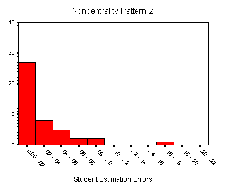Figure 2 (4.0K gif)

Figure 2. Histogram of Student Estimation Errors for Noncentrality Pattern 2.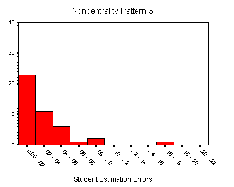Figure 3 (4.0K gif)

Figure 3. Histogram of Student Estimation Errors for Noncentrality Pattern 3.

23 The student results suggest that power estimates obtained from the Pearson and Hartley charts will, on average, show good agreement with exact power values (within 2%), but that students will frequently make moderate to severe estimation errors. Use of a computer program like SAS or Sample Power to estimate power alleviates this problem.

# 5. Recommendations

24 Our view is that students who are learning to estimate power are better served by using computer software designed for this task than by the more traditional Pearson and Hartley power charts. Computer programs produce power estimates that, given the values submitted, are exact and do not require students to visually separate curves or to interpolate. These programs also permit power to be estimated for a nominal Type I error rate of the user's choice. The use of software to estimate power opens up instructional opportunities that are not generally possible if power charts are used. For example, students could be asked to generate power curves across various parameter values to examine the consequences of changing effect sizes or sample sizes on power. A favorite exercise in our classes is to ask students to calculate power using SAS for published articles in their fields of study and to examine patterns in the estimated power of statistical tests. We think that this use of student time has more instructional value than that associated with learning to use the Pearson and Hartley power charts.

# References

Brewer, J. K. (1972), "On the Power of Statistical Tests in the American Educational Research Journal," American Educational Research Journal, 9(3), 391-401.

Cohen, J. (1962), "The Statistical Power of Abnormal-Social Psychological Research: A Review," Journal of Abnormal and Social Psychology, 65(3), 145-153.

----- (1988), Statistical Power Analysis for the Behavioral Sciences (2nd ed.), New York: Academic Press.

Dayton, C. M., Schafer, W. D., and Rogers, B. G. (1973), "On Appropriate Uses and Interpretations of Power Analysis: A Comment," American Educational Research Journal, 10(3), 231-234.

Glass, G. V., and Hopkins, B. K. (1984), Statistical Methods in Education and Psychology (2nd ed.), New York: Prentice-Hall.

Greenwald, A. G. (1975), "Consequences of Prejudice Against the Null Hypothesis," Psychological Bulletin, 82, 1-20.

Hays, W. L. (1994), Statistics (5th ed.), Fort Worth, TX: Holt, Rinehart, & Winston.

Keppel, G. (1991), Design and Analysis: A Researcher's Handbook (3rd ed.), Englewood Cliffs, NJ: Prentice-Hall.

Kirk, R. E. (1995), Experimental Design: Procedures for the Behavioral Sciences (3rd ed.), Pacific Grove, CA: Brooks/Cole.

Maxwell, S. E., and Delaney, H. D. (1990), Designing Experiments and Analyzing Data: A Model Comparison Perspective, Belmont, CA: Wadsworth.

Moore, D. S., and McCabe, G. P. (1993), Introduction to the Practice of Statistics (2nd ed.), New York: W. H. Freeman.

Odeh, R. E., and Fox, M. (1991), Sample Size Choice: Charts for Experiments with Linear Models (2nd ed.), New York: Marcel Dekker.

Pearson, E. S., Hartley, H. O. (1951), "Charts of the Power Function for Analysis of Variance Tests, Derived from the Non-Central F-distribution," Biometrica, 38, 112-130.

SAS Institute Inc. (1990), SAS/IML Software: Usage and Reference, Version 6 (1st ed.), Cary, NC: Author.

Serlin, R. C., and Lapsley, D. K. (1985), "Rationality in Psychological Research: The Good-Enough Principle," American Psychologist, 40, 73-83.

Tang, P. C. (1938), "The Power Function of the Analysis of Variance Tests with Tables and Illustrations of Their Use," Statistics Research Memorandum, 2, 126-149.

Thomas, L., and Krebs, C. J. (1997), "A Review of Statistical Power Analysis Software," Bulletin of the Ecological Society of America, 78, 128-139.

Zumbo, B. D., and Hubley, A. M. (1998), "A Note on Misconceptions Concerning Prospective and Retrospective Power," The Statistician, 47, 385-388.

Guido G. Gatti gggst3@vms.cis.pitt.edu
Michael Harwell harwell@vms.cis.pitt.edu